# UFR 4-10 Description

(diff) ← Older revision | Latest revision (diff) | Newer revision → (diff)

# Description

## Introduction

Buoyant flows in simple cavities represent one further level of complexity compared with simpler unconstrained buoyant flows such as wall layers and plumes. Buoyant flows in cavities may contain both wall flows and plumes as sub-elements but it can no longer be assumed that these have no effect on their external flow. In the present case only the specific subclass of two-dimensional rectangular cavities with heated vertical walls and insulated (adiabatic) horizontal walls will be considered here as these already offer a variety of interesting phenomena. There are a wide variety of alternative buoyant cavity arrangements which have been studied both experimentally and computationally however these are beyond the scope of this review.

This type of underlying flow regime is of practical interest for example in thermal insulation, heating and ventilation of buildings, smoke spread from fires, the behaviour of solar collectors and the cooling of electronic equipment. Useful reviews of the general physics of buoyant flows in are given in standard texts and detailed reviews of buoyant cavity flows are given for example by Bejan, Gebhart et al. and Ostrach.

Figure 1 shows a schematic diagram of the general arrangement considered here, with the side walls maintained at different uniform constant temperatures, Th and Tc, and the upper and lower horizontal walls assumed to be adiabatic. The cavity has a width W and height H and the aspect ratio, H/W, is of significance in defining the various possible flow regimes. Cavities with H/W > 1 are generally termed “tall” and those with H/W < 1 “shallow”.

The flow is characterized by the Prandtl, Grashof and Rayleigh numbers:

 $\displaystyle {Ra=GrPr={\frac {g\beta H^{3}\Delta T}{\nu ^{2}}}Pr}$where g is the magnitude of the gravitational acceleration, H the cavity height, ΔT the hot to cold wall temperature difference and ν the kinematic viscosity. The Grashof number represents the ratio of buoyancy to viscous forces, giving a measure of the stability of the flow regime, with low Grashof (or Rayleigh) numbers typified by stable laminar flow and high Grashof (or Rayleigh) numbers by fully turbulent flow.

A further relevant parameter is the non-dimensional temperature difference, $\left.\theta \right.$:

 $\displaystyle {\theta ={\frac {\Delta T}{T_{c}+T_{k}}}}$which gives an indication of the size of the temperature difference relative to the mean temperature. For low values of $\left.\theta \right.$, for example $\left.\theta <0.05\right.$, the Boussinesq approximation can be considered valid for most applications (depending upon the accuracy required) whereas, as $\left.\theta \right.$increases, the variation of density must be accounted for in all terms if an accurate solution is to be obtained.

Of particular interest is the heat transfer rate across the cavity, usually expressed as a mean Nusselt number:

 $\displaystyle {{\overline {Nu}}={\frac {Q}{Q_{c}}}}$where Q is the total heat transfer rate and Qc the heat transfer rate due to pure conduction:

 $\displaystyle {Q_{c}=k\Delta T\left({\frac {H}{W}}\right)}$in which k is the thermal conductivity.

Bejan defines four limiting flow regimes based on the cavity aspect ratio and Rayleigh number:

1. Conduction limit: close to linear variation of temperature across cavity with slow single-cell recirculation and mean Nusselt number close to unity, for any aspect ratio with very low Ra.
2. Tall-enclosure limit: for large aspect ratio the heat transfer is dominated by conduction and the mean Nu is again close to one.
3. High-Ra limit (boundary-layer regime): distinct thermal and hydrodynamic boundary layers are observed along the vertical walls while the core of the cavity is relatively stagnant and thermally stratified.
4. Shallow-enclosure limit: marked by heat exchange between the hot and cold horizontal streams which now have extensive contact and can act as insulation between the vertical walls.

In the following, first the case of laminar flow will be considered which provides a useful test case for evaluating numerical methods with combined fluid flow and heat transfer and for which a number of numerical benchmarks are available. In the third section turbulent flow cases are considered for which experimental data are available and one particular case is identified as a reference.

## Review of UFR studies and choice of test case

LAMINAR FLOW

The laminar, two-dimensional, buoyancy-driven flow in a square cavity has become a standard test case for numerical methods predicting combined fluid flow and heat transfer. As shown in Figure 1, the basic arrangement consists of a closed square cavity with uniform fixed temperatures imposed on the two vertical walls and adiabatic conditions applied on the upper and lower horizontal walls. This creates a recirculating flow driven by the temperature difference. Steady laminar solutions for the square cavity with air as the working fluid (Pr=0.7) can be obtained up to at least Ra=106 though the flow becomes increasingly unstable at higher Ra values.

A number of numerical studies of laminar buoyant flows in simple enclosures are available for comparison, including those by Chenoweth and Paolucci , Demird&#x009E;ić et al. , De Vahl Davis , Hortmann et al. , Janssen and Henkes , Le Quéré  and Winters , in both the Boussinesq and non-Boussinesq regimes.

For the three cases considered by Hortmann et al.  Pr=0.71 and Ra=104, 105 and 106 respectively. Ra is varied using the cavity dimensions, keeping the other properties constant, as summarized in Table 1 which includes also the predicted mean and peak Nusselt numbers. For these cases the non-dimensional temperature difference θ=0.018, well within the Boussinesq regime. The results presented in  were computed using a cell-centred finite-volume method on a series of refined Cartesian meshes going up to 320x320 in size. Certain values, including the peak and mean Nusselt numbers, were then calculated using Richardson extrapolation to achieve the proposed reference values. The values given by Hortmann et al. differ slightly from the earlier reference proposed by De Vahl Davis  (extrapolated from an 81x81 mesh) but are very close to Le Quéré's results , computed using a pseudo-spectral method, as well as those of Janssen and Henkes .

 Ra ρ μ x 105 β Pr g Tc Th L=H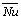Numax 104 1.19 1.8 0.00341 0.71 9.81 2 12 0.021277 2.24475 3.53087 105 1.19 1.8 0.00341 0.71 9.81 2 12 0.045841 4.52164 7.72013 106 1.19 1.8 0.00341 0.71 9.81 2 12 0.098761 8.82513 17.536

Figures 2 to 4 show velocity vectors and contours of temperature for the three Rayleigh numbers considered by Hortmann et al. . The results were calculated on uniformly-triangulated Cartesian meshes using a system residual-distribution approach for convection and the Galerkin finite-element method for diffusion . Though all of the cases fall within the boundary-layer regime, as defined by Bejan , large differences can be observed. At Ra=104 the temperature contours are not yet severely distorted from their straight pure-conduction form and there is no strong vertical stratification. In contrast, for Ra=106, strong temperature gradients can be observed normal to the vertical walls and in the central region there is noticeable vertical stratification. Coupled to this, the velocity field now consists of two well-defined boundary layers on the vertical walls with a region of near-stagnant fluid in the centre of the cavity.

Another key feature which can be observed is the antisymmetric nature of the velocity and temperature fields, typical of flows in the Boussinesq regime . The temperature and momentum boundary layers on the two vertical walls are identical in form, differing only in the direction of movement and the orientation of the gradients. This is not the case for non-Boussinesq flows where the layer on the hot wall can become considerably thicker than that on the cold wall.

When the size of the temperature difference in a buoyant flow becomes significant relative to the average temperature, i.e. for large values of θ, the Boussinesq approximation ceases to be valid and the variation of density must be accounted for in all terms and equations. In this section the application of the present method to such non-Boussinesq flows is demonstrated.

Paillère and Le Quéré proposed four cases for a workshop on natural convection with large temperature differences  which took place in January 2000 and for which results were submitted using a number of different methods. All four cases relate to flow in a square cavity for Ra=106 with an arrangement as shown in Figure 1. The cases all have the same uniform initial thermodynamic conditions: p0=101325Pa and Tref=600K and the fluid is taken to be an ideal gas with the properties of air (gas constant 287J/(kgK), γ=1.4 and Pr=0.71).

Unlike the Boussinesq case, the initial conditions are significant here as they define the mass of air contained in the cavity, which must remain unchanged during the solution procedure. It is interesting to note that a number of computational results initially submitted to the workshop did not succeed in conserving mass. The cases used either Sutherland's law to define a variable viscosity and thermal conductivity or constant transport properties calculated at Tref.

The boundary conditions, with hot and cold fixed-temperatures on the two vertical walls and adiabatic conditions on the horizontal walls, are the same as for the Boussinesq cavity flow, shown in Figure 1. Four different relative temperature differences are considered: θ=0.01, 0.2, 0.4 and 0.6 with the wall temperatures given by: Th=(1+θ)Tref and Tc=(1-θ)Tref.

In the boundary-layer regime the constraints of continuity mean that the mass flows across the layers at equivalent locations on the hot and cold walls must be approximately equal. As the temperature difference increases the low-density layer on the hot wall tends to become thicker and the high-density layer on the cold wall thinner to preserve this equality of mass flow. This

effect is only slightly visible for θ=0.2 but can be observed strongly at θ=0.6.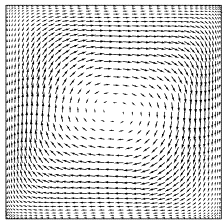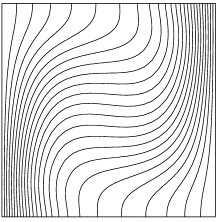Figure 2: velocity vectors (left) and temperature contours (right) for laminar buoyancy-driven flow in a square cavity at Ra=104.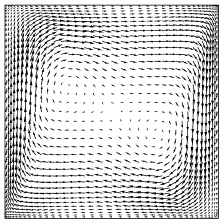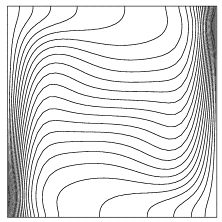Figure 3: velocity vectors (left) and temperature contours (right) for laminar buoyancy-driven flow in a square cavity at Ra=105.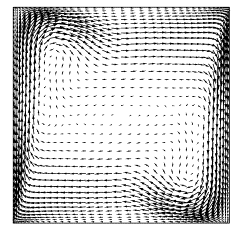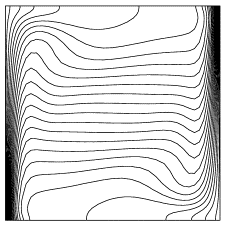Figure 4: velocity vectors (left) and temperature contours (right) for laminar buoyancy-driven flow in a square cavity at Ra=106.

TURBULENT FLOW

The relative paucity of definitive reference cases for turbulent two-dimensional buoyancy-driven flows in simple cavities is indicative of the experimental difficulties associated with creating such flows in the laboratory. These difficulties relate in particular to the creation of a truly two-dimensional mean flow and to the clear specification of thermal boundary conditions. The available DNS data for such flows also appears to be quite limited at this time though some data sets are available for flows in vertical slots, for example that of Boudjemadi et al.  and Versteegh and Nieuwstadt . The level of turbulence in the cavity is governed by the Rayleigh number and the aspect ratio (H/W) of the cavity, with higher values of Ra and H/W tending to lead to higher turbulence levels.

The studies of Cheesewright et al. [9-11] have been used quite widely as a reference for numerical simulations as they provide both hydrodynamic and thermal data. These experiments were carried out using an air-filled cavity of aspect ratio 5:1 at a Rayleigh number of 4 x 1010 using laser-doppler velocimetry. Unfortunately the results suffer from significant end effects associated with heat transfer through the vertical side walls and the top wall. Betts et al. have also conducted a series of experiments on tall air-filled cavities [3-5, 13] of aspect ratio 28.6:1 and with Ra around 106. One of these is chosen here as the reference case and is described in detail below. Tian et al. [60, 61] carried out measurements in a square air-filled cavity at Ra=1.58 x 109, attempting to rectify the weakness of earlier experiments and presenting both temperature and velocity data. Other experimental studies include those of Elder , Giel and Schmidt , Lankhorst et al. , Opstelten et al.  and Mergui and Penot . In the case of low-aspect-ratio cavities, such as those of Tian et al. [60, 61] and Mergui and Penot , it is difficult to achieve significant turbulence levels, while in tall, high-aspect-ratio cavities, such as those of Betts et al. [3-5, 13], higher turbulence levels can be achieved but the flow tends towards that between two infinite vertical heated plates.

There have also been a number of experimental studies carried out on flows in rectangular cavities with various arrangements of internal partitions which can lead to interesting flow behaviour. Two examples are the work of Nansteel and Greif  and Lin and Bejan .